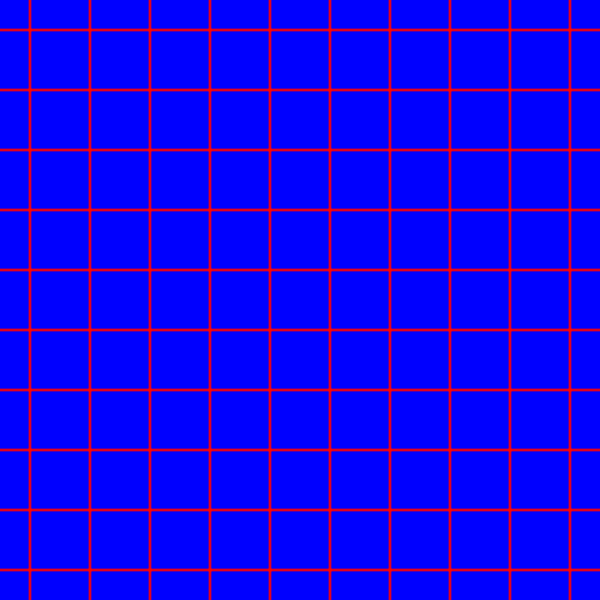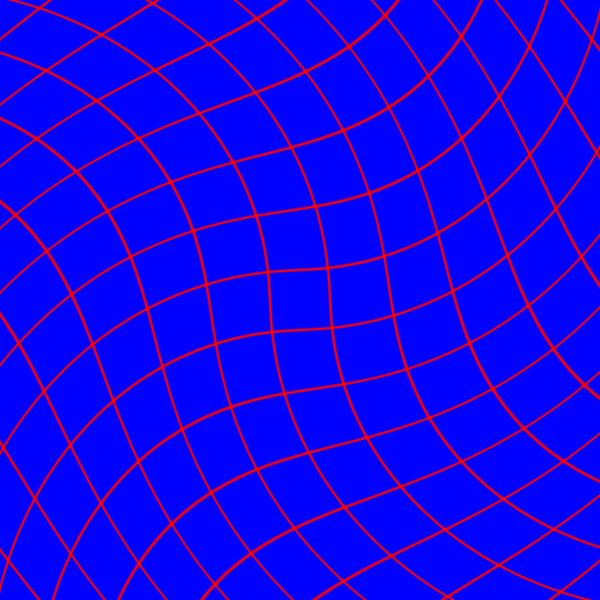# Warp the coordinate space

## A bit of theory about the OpenGL graphic pipeline

In the OpenGL graphics pipeline, the fragment processor is the programmable unit that operates on fragment values. Shaders that are intended to run in this processor are called fragment shaders. Refer to the book OpenGL Shading Language by R.J. Rost and B. Licea-Jane for a deep understanding of the OpenGL graphics pipeline.

The fragment values are computed between the vertex processor and the fragment processor, and they are made available to the fragment shader through special input variables. The primary inputs to the fragment shader are the interpolated varying variables.

The window coordinate position of the fragment is communicated through the special input varying variable `vec4 gl_FragCoord`. This window position value is the result of the fixed functionality that interpolates primitives after vertex processing to generate fragments.

## A simple exercise: warp an image by transforming the space coordinates

The fragment shader is executed on the fragment processor and produces output values based on the input values that are provided. One of the user-defined output variables is the `vec4 gl_FragColor` variable, which assigns the RGB colour to the fragment. There is a notebook of this exercise, have look at https://observablehq.com/@riccardoscalco/warp-glsl-coordinate-space.

A fragment shader cannot change the fragment position `gl_FragCoord`, its task is to define the `gl_FragColor` variable as a function of `gl_FragCoord`.

In the next examples, the fragment position is expressed by a variable `vec2 p` with values in `[0, 1]`. The exercise we are going to follow is based on two functions. The first function defines the fragment colour taking the fragment position as the argument: `vec4 colour = f(p)`. The second function `vec2 g(p)` warps the coordinate space defined by the values of `p`.

The composition of `f` and `g` will output a distorted version of the original drawing: `vec4 warpedColor = f(g(p))`.

## Draw a lattice on the canvas

To better visualize the space transformation, the function `f` above implements a lattice.

Listing 1 shows the implementation of the `smoothSquare` function, which produces a smooth square function along a given direction, with peaks nearly `2 * delta` wide. The `lattice` function is defined as the sum of two `smoothSquare` drawn at two perpendicular directions: `p.x` and `p.y`.Listing 1. Function lattice draws a lattice on the space. Note that the number of cells per unit of area can be increased with a scaling of the space coordinates, for example with p → p * s, where s is the scaling factor (see listing 2 below).

## Warp the coordinate space with a rotation around a point

For the sake of convenience, we are going to implement the space transformation `g` as a rotation around the canvas centre.

Function `translateAndScale` in listing 2 scales the vector `p` of a factor `s` and translates `p` so that the point `p = vec2(0., 0.)` would be at the centre of the canvas.

Function `rotate2D` in listing 3 implements a two-dimensional rotation of an angle `alpha`.

Function `warp` applies the rotation to the point `p`, with an angle `alpha` that is proportional to the distance of `p` from the canvas centre.Listing 3. Function warp rotates the two-dimensional vector p of a variable angle. The angle alpha is a function of the distance between the canvas centre and the vector p.

## Distort the lattice with function composition

Eventually, we make use of function composition to warp the lattice drawn in listing 1. The function `lattice(warp(p))` results from the composition of functions `warp` and `lattice`.